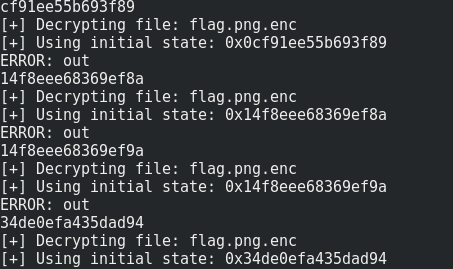This post spoils a CTF challenge … Don’t read if you want to try it !

This CTF was organized by the ANSSI to recruit potential members for the French ECSC CTF team. I managed to finish 7th in the senior category (21-25 years old).

## [+] Presentation

Analysez l’algorithme de chiffrement présent dans le fichier cipher.py et déchiffrez flag.png.enc.

## [+] Analysis

First, let’s try to understand how the algorithm works.
It is based on a randomly generated state. This state acts like the encryption key and is needed to decrypt a file.
For each bit of the cleartext, the algorithm generates a bit from the actual state and xor it with the cleartext bit.
After a bit generation, the state is updated : it loses his first bit, and a new final bit is appended. This final bit is computed with the current state.

``````
Our goal is to decrypt the given encrypted file without the secret key, or try to find the secret key.\

Here, we can take advantage from the fact that we know the clear text filetype (.png).\
Indeed, the first bytes of a PNG file doesn't change from a file to another one, so we can use this fact to retrieve the first bytes generated by the encryption algorithm with the random state.

PNG signature :
89 50 4E 47 0D 0A 1A 0A

Encrypted file's first bytes :
8D BC 08 06 0E CA 22 08

Xor between PNG signature and encrypted file's first bytes :
04 EC 46 41 03 c0 38 02

So, the 64 first generated bits are :\
``````

BASE = 00000100 11101100 01000110 01000001 00000011 11000000 00111000 00000010```

By simplifying the ‘Filtered’ function, we can say that :

``````state) ^ (state & state) ^ (state & state) ^ (state))```

This can be extended to the 15 following bits by neglecting the new bits appended at the end of the state since we don't use it for the moment.\
``````

BASE[i] = (0 ^ (state[0 + i] & state[16 + i]) ^ (state[0 + i] & state[32 + i] & state[48 + i]) ^ (state[16 + i] & state[32 + i] & state[48 + i]) ^ (state[16 + i] & state[32 + i]) ^ (state[16 + i] & state[48 + i]) ^ (state[16 + i]))```

For the 16 bits after, we can use the same formula with the 16 new bits appended at the end of the state.
So on, we proceed in the same way for all the bits that we extracted.
In this way, we can establish precise conditions that must be respected by the state we are looking for.

## [+] Resolution

We will now use a SAT-Solver like z3, which will help us to quickly identify the combinations which respect the conditions established by our previous findings.
You can find my solver script here.
The resulting possible combinations are writed in this file.

Now, we just need to try the remaining combinations with a simple bash script.After a few minutes, we have the flag !